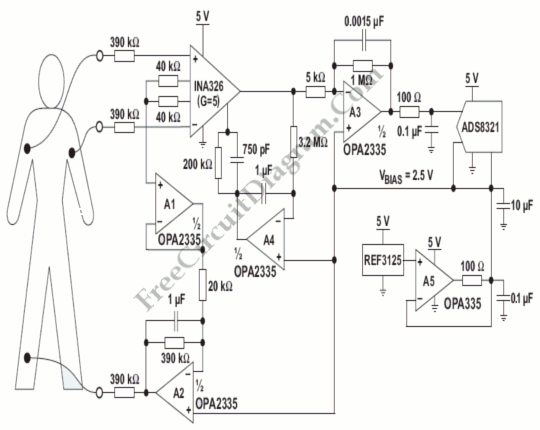## Fast Logarithmic Amplifier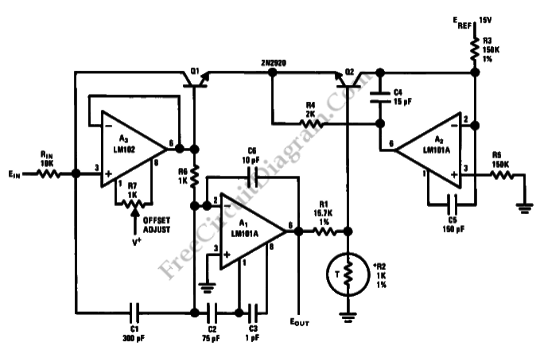This is Fast Logarithmic Amplifier circuit. Dynamic range of This circuit has is 80 dB. This circuit is configured to optimize speed rather than dynamic range. To allow the use of feed forward compensation1 on an LM101A operational amplifier, this circuit uses Transistor Q1 that is connected with diode. This compensation can increase the slew rate and extends the bandwidth […]

## Power Function Circuit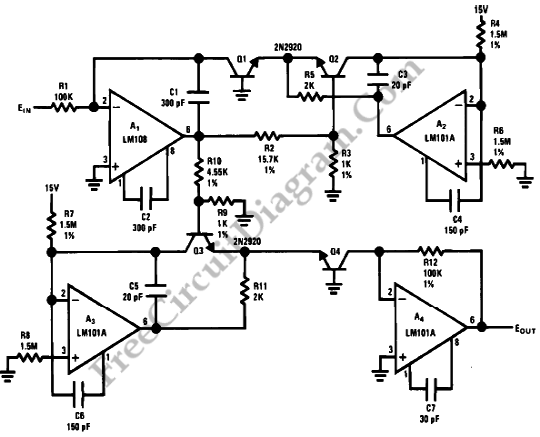We can generate easily many non-linear functions such as X^1/2, X^2, X^3, 1/X, XY and X/Y using logs. Division becomes subtraction, multiplication becomes addition and powers become gain coefficients of log terms. The circuit diagram below shows a circuit whose output is the cube of the input. Actually, by changing the values of R9 and R10 in accordance with the […]

## Log Converter with 100dB Dynamic Range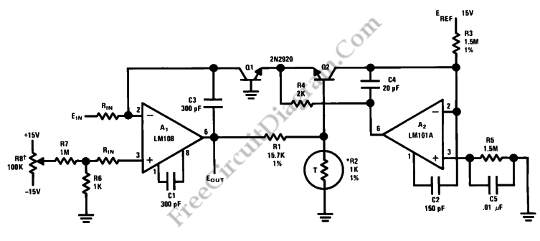This is a circuit of Log Converter with 100dB Dynamic Range. This circuit can give a a logarithmic output voltage for a linear input current. This circuit use an LM108 operational amplifier with Transistor Q1 as non-linear feedback element.  R1 and R2 are used as divider. Negative feedback is applied to the emitter base junction of Q2 and the emitter […]

## Frequency Doubler with 4011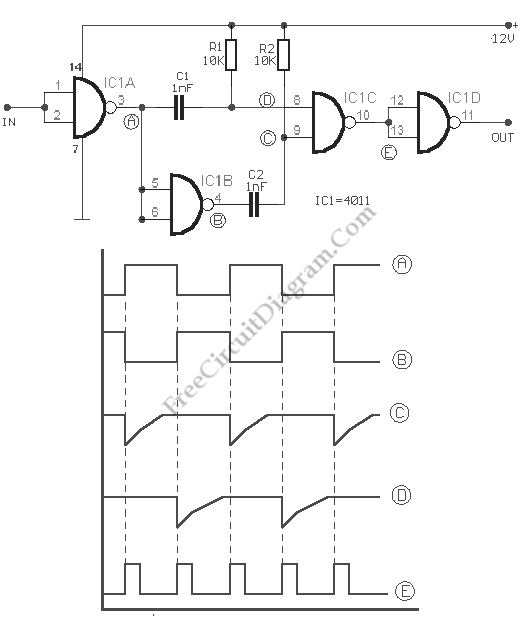This is a circuit of Frequency Doubler using 4011. This circuit uses one CMOS quad, two input NAND gate package type 4011. This circuit consist of two differentiating networks R1/C1, R2/C2, an inverter IC1B and NAND gate IC1A, IC1D, IC1C and  function as input and output buffers. Figure below shows the schematic diagram of the circuit and the pulses in […]

## Audio Speech Compressor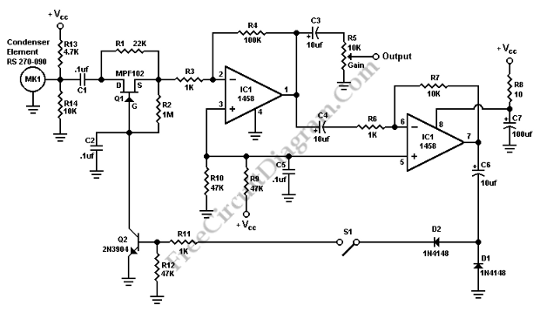There are many low power SSB rigs and kits don’t have any real speech processor circuitry, although there is a built in speech processor in most modern HF. Speech processing is an important thing, when the SSB is operated at low power to get through the QRM. Speech processing can be done by this circuit. This is a simple and […]

## Analog Multiplier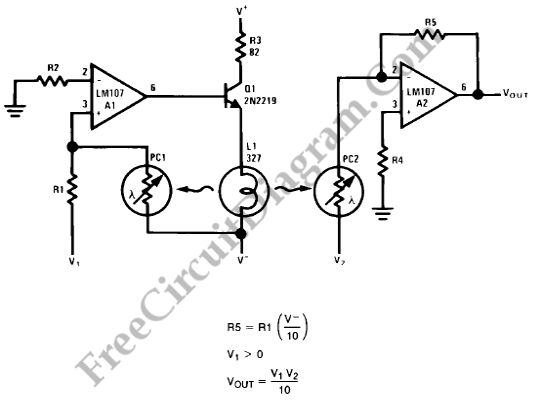The figure below shows a simple embodiment of the analog multiplier. This circuit provides three quadrant analog multiplication which is relatively temperature insensitive and which is not subject to the bias current errors which is plague most multipliers and circumvents many of the problems associated with the log-antilog circuit. By considering A2 as a controlled gain amplifier, amplifying V2, whose […]